# 9.1: Molecular Shapes

$$\newcommand{\vecs}{\overset { \rightharpoonup} {\mathbf{#1}} }$$ $$\newcommand{\vecd}{\overset{-\!-\!\rightharpoonup}{\vphantom{a}\smash {#1}}}$$$$\newcommand{\id}{\mathrm{id}}$$ $$\newcommand{\Span}{\mathrm{span}}$$ $$\newcommand{\kernel}{\mathrm{null}\,}$$ $$\newcommand{\range}{\mathrm{range}\,}$$ $$\newcommand{\RealPart}{\mathrm{Re}}$$ $$\newcommand{\ImaginaryPart}{\mathrm{Im}}$$ $$\newcommand{\Argument}{\mathrm{Arg}}$$ $$\newcommand{\norm}{\| #1 \|}$$ $$\newcommand{\inner}{\langle #1, #2 \rangle}$$ $$\newcommand{\Span}{\mathrm{span}}$$ $$\newcommand{\id}{\mathrm{id}}$$ $$\newcommand{\Span}{\mathrm{span}}$$ $$\newcommand{\kernel}{\mathrm{null}\,}$$ $$\newcommand{\range}{\mathrm{range}\,}$$ $$\newcommand{\RealPart}{\mathrm{Re}}$$ $$\newcommand{\ImaginaryPart}{\mathrm{Im}}$$ $$\newcommand{\Argument}{\mathrm{Arg}}$$ $$\newcommand{\norm}{\| #1 \|}$$ $$\newcommand{\inner}{\langle #1, #2 \rangle}$$ $$\newcommand{\Span}{\mathrm{span}}$$$$\newcommand{\AA}{\unicode[.8,0]{x212B}}$$

The Lewis electron-pair approach described previously can be used to predict the number and types of bonds between the atoms in a substance, and it indicates which atoms have lone pairs of electrons. This approach gives no information about the actual arrangement of atoms in space, however.

## Molecular Geometry

The specific three dimensional arrangement of atoms in molecules is referred to as molecular geometry. We also define molecular geometry as the positions of the atomic nuclei in a molecule. There are various instrumental techniques such as X-Ray crystallography and other experimental techniques which can be used to tell us where the atoms are located in a molecule. Using advanced techniques, very complicated structures for proteins, enzymes, DNA, and RNA have been determined. Molecular geometry is associated with the chemistry of vision, smell, taste, drug reactions, and enzyme controlled reactions to name a few.

##### Example $$\PageIndex{1}$$: Carbon Tetrachloride

The Lewis structure of carbon tetrachloride provides information about connectivities, provides information about valence orbitals, and provides information about bond character.However, the Lewis structure provides no information about the shape of the molecule, which is defined by the bond angles and the bond lengths. For carbon tetrachloride, each C-Cl bond length is 1.78Å and each Cl-C-Cl bond angle is 109.5°. Hence, carbon tetrachloride is tetrahedral in structure: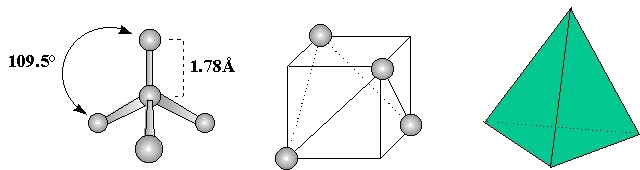## Molecular Geometries of $$AB_n$$ molecules

Molecular geometry is associated with the specific orientation of bonding atoms. A careful analysis of electron distributions in orbitals will usually result in correct molecular geometry determinations. In addition, the simple writing of Lewis diagrams can also provide important clues for the determination of molecular geometry. Molecular shapes, or geometries, are critical to molecular recognition and function. Table $$\PageIndex{1}$$ shows some examples of geometries where a central atom $$A$$ is bonded to two or more $$X$$ atoms. As indicated in several of the geometries below, non-bonding electrons $$E$$ can strongly influence the molecular geometry of the molecule; this is discussed in more details in VSEPR Model" data-cke-saved-href="/Bookshelves/General_Chemistry/Map:_Chemistry_-_The_Central_Science_(Brown_et_al.)/09:_Molecular_Geometry_and_Bonding_Theories/9.02:_The_VSEPR_Model" href="/Bookshelves/General_Chemistry/Map:_Chemistry_-_The_Central_Science_(Brown_et_al.)/09:_Molecular_Geometry_and_Bonding_Theories/9.02:_The_VSEPR_Model" data-quail-id="26">Section 9.2.

Table $$\PageIndex{1}$$:
6 5 4 3 2
AX6octahedral
AX5trigonal bipyramidal
AX4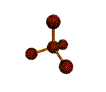tetrahedral
AX3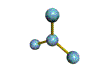trigonal planar
AX2linear
1 lone pair of electrons
AX5E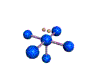square pyramidal
AX4E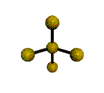distorted tetrahedron
AX3Epyramidal
AX2Enonlinear
AXElinear
2 lone pairs of electrons
AX4E2square planar
AX3E2T-shaped
AX2E2bent
AXE2linear

These structures can generally be predicted, when A is a nonmetal, using the "valence-shell electron-pair repulsion model (VSEPR) discussed in the next section.